Definition of Morse Potential

What is the Morse Potential?

Two atoms that can form a bond will do so to create a diatomic molecule when they approach each other closely. This situation is possible provided the potential energy of the product is lower than that of the reactants. We describe the energy minimum with a potential energy curve, called a potential well.

The potential energy, V(R), of a diatomic molecule can be described by the Morse potential: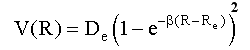where De is the well depth, R is internuclear distance, Re is the equilibrium internuclear distance (bond length), and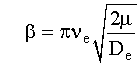νe is the vibrational constant and μ is the reduced mass.

The following figure shows the ground state potential well of the H2 molecule.

The curve is calculated from the Morse potential and the energy levels are calculated using the harmonic oscillator model with the first anharmonic correction.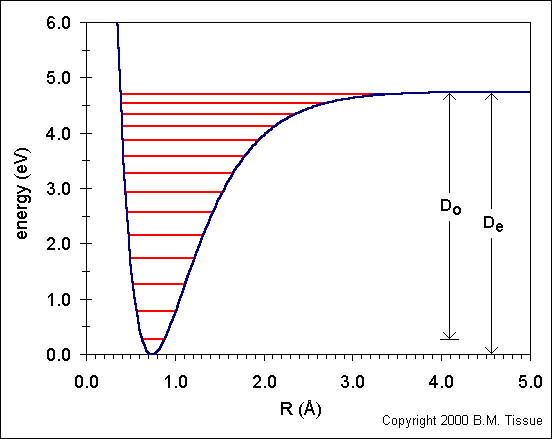Do is the dissociation energy, which is slightly different from the well depth, De. The vibrational energy levels in this plot are calculated using the harmonic oscillator model: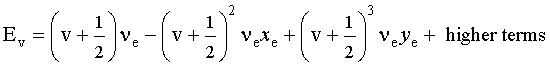where v is the vibrational quantum number, v = 0,1,2,..., and xe and ye are the first and second anharmonicity constants, respectively. The v = 0 level is the vibrational ground state.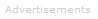Search the Dictionary for More Terms